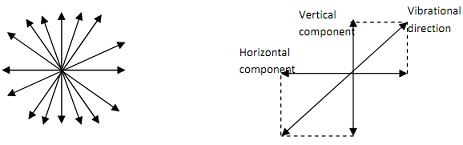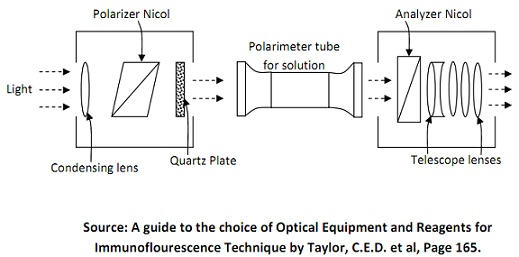#### Polarimetry

Introduction

Polarimetry is the technique of analysis that is based on the ability of a specified compound in solution to rotate a plane polarized light to an assured direction. A normal light beam consists of waves oscillating in random directions. Each of the vibrational directions can be determined into 2 equally perpendicular directions. But the real vibrational direction is the vector sum of the two components.If the ordinary light is collimated and passed through a crystal which allows only one vibrational orientation to be transmitted, the transmitted light is said to be plane polarized. Many natural crystals generate polarized light, but it is most conveniently attained through commercially accessible Polaroid materials.

Definition

An instrument utilized in measuring the optical rotation of a material in solution is termed a polarimeter and the phenomenon, polarimetry.

Basic Principle of Polarimetry

Several compounds in solution have the power of rotating the plane polarized light to an indeed direction. These compounds are said to be optically active and, in the case of organic compounds, are found to have one or more asymmetric carbon atoms inside the molecule. An asymmetric carbon atom is one by 4 different groups connected to it. The degree or direction of the rotation is either to the right (termed dextrorotatory, +) or to the left (described laevorotatory, -).

The extent of the degree of rotation of the plane polarized light is dependent on the:-

• Nature of the compound;
• Nature of the solvent;
• Depth of the solution through that the light passes;
• Concentration of the solution;
• Temperature; and
• Wavelength of the light utilized.

The wavelength of the light generally employed is that of sodium D line (589.3 nm), and the temperature is kept steady at 20 of 250C inside the instrument.

Mode of Operation of a Polarimeter

Before operation, the analyzer prism of the polarimeter is turned to build both halves of the field of examination uniformly bright; the circular scale reading for this position of the analyzer prism is reminded as its zero position. The polarimeter tube is then sealed through the experimental solution, the jacket around the tube is filled by water at a specified temperature and the solution is permitted to stand in the tube for several times to achieve that temperature. On passing the monochromatic light once more through the polarimeter, the 2 halves of the field of vision now look unequally illuminated due to the rotation of the plane polarized light via the solution in the tube. The analyzer prism is resolved until both halves look uniformly bright once more. The difference in the circular scale reading between the final position of the analyzer prism and its zero position recorded at 1st provides the angle of rotation of the polarized light.

Instrumentation

Optical rotation is generally calculated through a polarimeter or polariscope and the phenomenon is identified Polarimetry. The polarimeter consists of a long hollow barrel carrying at its reverse ends, a fixed polarizer Nicol prism and a rotating analyzer Nicol prism correspondingly. A hollow polarimeter tube, encircled through a jacket, can be situated within the barrel between the 2 prisms. This tube of a specified length is intended for carrying the solution under investigation whilst the jacket around it might be sealed through water at a specified temperature. Between the polarizer prism and the polarimeter tube, a quartz plate is located for obscuring half the field of vision. Beyond the analyzer prism are situated the telescopic eyepiece lenses. That end of the polarimeter barrel carries a fixed circular scale regulated in degrees radian, the position of the analyzer prism might be read on this scale. A schematic diagram of a polarimeter is specified below:Fig: A Polarimeter

There are 3 necessary parts:

• The polarizer (a lens and nicol prism assembly to polarize the incident light)
• The polarimeter tube (a thick-walled glass tube by a screw on both ends incorporating optical glass windows) that encloses the solution under examination.
• The analyzer (a nicol prism, and eye piece assembly for crossing the plane of the emergent polarized light) that is filled by a degree scale and pointer.

Uses of Polarimeter

Below are several of utilizes of the instrument:

• From the angle of rotation "a" of the polarized light computed through the polarimeter, the length, l, of the solution column in the polarimeter tube and the concentration C of the solution, the exact rotation α of the solute might be calculated via the formula specified below:

[α]D25 = α / l*C

• An optically energetic substance might be identified in a solution of a recognized concentration through measuring its optical rotation.
• From the optical rotation of a mixed solution of 2 substances having recognized precise rotations (for example sucrose and glucose), their relevant concentrations in that solution can be estimated.
• If α is already recognized for the solute, its concentration C in the solution can be computed using the estimated angle of rotation, a, and the length, l, of the solution column.

Tutorsglobe: A way to secure high grade in your curriculum (Online Tutoring)